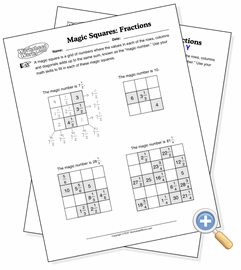# Fraction Magic SquaresDevelop process of elimination skills with fractions

This is a standard magic square that has been modified to use fractions instead of integers.

A magic square is a grid of numbers where the values in each of the rows, columns and diagonals adds up to the same sum, known as the “magic number.” In this puzzle, the magic number is given but many of the cells are left empty. There are just enough cells filled with values that one can use deduction to figure out what the other cells contain.

A magic square worksheet contains three or four puzzles (it is up to you if the first one serves as an example), each one a row and column larger than the last. Select the starting cell size to determine how large they get.